You've successfully subscribed to Better Data Science
Welcome back! You've successfully signed in

# Are The New M1 Macbooks Any Good for Data Science? Let’s Find OutThe new Intel-free Macbooks have been around for some time now. Naturally, I couldn’t resist and decided to buy one. What follows is a comparison between the 2019 Intel-based MBP and the new one in programming and data science tasks.

If I had to describe the new M1 chip in a single word, I would be this one — amazing. Continue reading for a more detailed description.

Data science aside, this thing is revolutionary. It runs several times faster than my 2019 MBP while remaining completely silent. I’ve run multiple CPU exhaustive tasks, and the fans haven’t kicked in even once. And, of course, the battery life. It’s incredible — 14 hours of medium to heavy use without a problem.

But let’s focus on the benchmarks. There are five in total:

• CPU and GPU benchmark
• Performance test — Pure Python
• Performance test — Numpy
• Performance test — Pandas
• Performance test — Scikit-Learn

## Important notes

If you’re reading this article, I’m assuming you’re considering if the new Macbooks are worth it for data science. They aren’t “deep learning workstations” for sure, but they don’t cost that much, to begin with.

All comparisons throughout the article are made between two Macbook Pros:

• 2019 Macbook Pro (i5–8257U @ 1.40 GHz/8 GB LPDDR3/Iris Plus 645 1536 MB) — referred to as Intel MBP 13-inch 2019
• 2020 M1 Macbook Pro (M1 @ 3.19 GHz/8GB) — referred to as M1 MBP 13-inch 2020

Not all libraries are compatible yet on the new M1 chip. I had no problem configuring Numpy and TensorFlow, but Pandas and Scikit-Learn can’t run natively yet — at least I haven’t found working versions.

The only working solution was to install these two through Anaconda. It still runs through a Rosseta 2 emulator, so it’s a bit slower than native.

The test you’ll see aren’t “scientific” in any way, shape or form. They only compare runtimes in a different set of programming and data science tasks between the mentioned machines.

## CPU and GPU benchmark

Let’s start with the basic CPU and GPU benchmarks first. Geekbench 5 was used for the tests, and you can see the results below: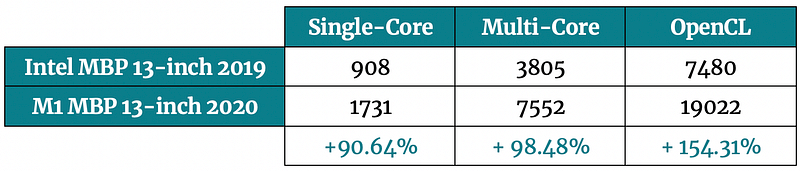Image 1 — Geekbench comparison (CPU and GPU) (image by author)

The results speak for themselves. M1 chip demolished Intel chip in my 2019 Mac. This benchmark only measures overall machine performance and isn’t 100% relevant for data science benchmarks you’ll see later.

Still, things look promising.

## Performance test — Pure Python

Here’s a list of tasks performed in this benchmark:

• Create a list `l` containing 100,000,000 random integers between 100 and 999
• Square every item in `l`
• Take a square root of every item in `l`
• Multiply corresponding squares and square roots
• Divide corresponding squares and square roots
• Perform an integer division of corresponding squares and square roots

The test was made only with built-in Python libraries, so Numpy wasn’t allowed. You can see the Numpy benchmark in the next section.

Here’s the code snippet for the test:

``````import random

time_start = datetime.now()

l = [random.randrange(100, 999) for i in range(100000000)]

squared = [x**2 for x in l]
sqrt = [x**0.5 for x in l]
mul = [x * y for x, y in zip(squared, sqrt)]
div = [x / y for x, y in zip(squared, sqrt)]
int_div = [x // y for x, y in zip(squared, sqrt)]

time_end = datetime.now()
print(f'TOTAL TIME = {(time_end - time_start).seconds} seconds')``````

And here are the results: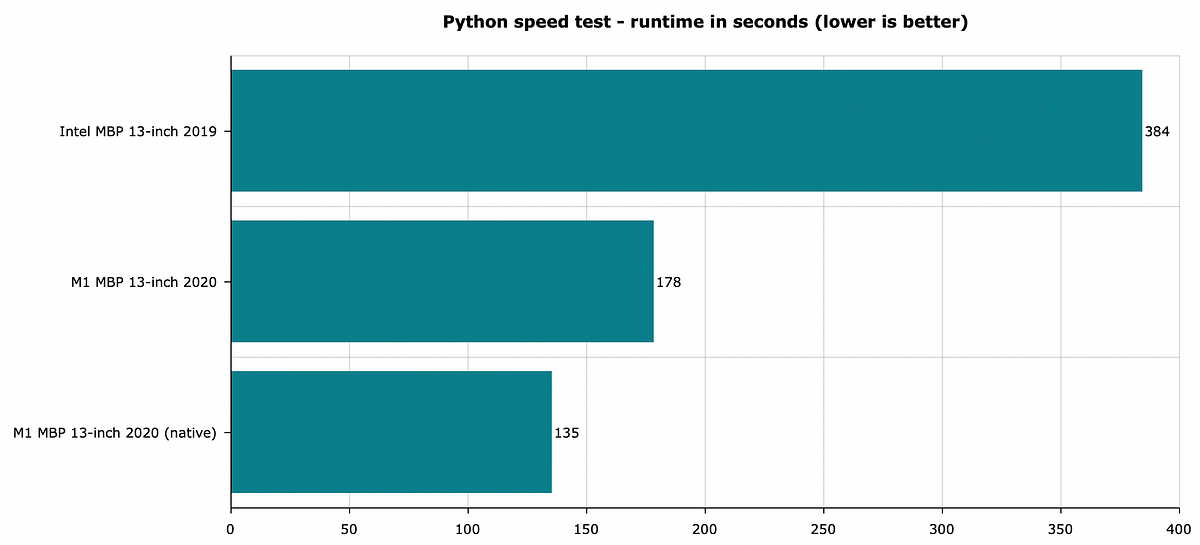Image 2 — Python speed test — lower is better (image by author)

As you can see, running Python on M1 Mac through Anaconda (and Rosseta 2 emulator) decreased the runtime by 196 seconds. It’s best to run Python natively, as this further reduces the runtime by 43 seconds.

To conclude — Python is approximately three times faster when run natively on a new M1 chip, at least per this benchmark.

## Performance test — Numpy

Here’s a list of tasks performed in this benchmark:

• Matrix multiplication
• Vector multiplication
• Singular Value Decomposition
• Cholesky Decomposition
• Eigendecomposition

The original benchmark script was taken from Markus Beuckelmann on Github, and modified slightly, so both start and end time is captured. Here’s how the script looks like:

``````# SOURCE: https://gist.github.com/markus-beuckelmann/8bc25531b11158431a5b09a45abd6276

import numpy as np
from time import time
from datetime import datetime

start_time = datetime.now()

# Let's take the randomness out of random numbers (for reproducibility)
np.random.seed(0)

size = 4096
A, B = np.random.random((size, size)), np.random.random((size, size))
C, D = np.random.random((size * 128,)), np.random.random((size * 128,))
E = np.random.random((int(size / 2), int(size / 4)))
F = np.random.random((int(size / 2), int(size / 2)))
F = np.dot(F, F.T)
G = np.random.random((int(size / 2), int(size / 2)))

# Matrix multiplication
N = 20
t = time()
for i in range(N):
np.dot(A, B)
delta = time() - t
print('Dotted two %dx%d matrices in %0.2f s.' % (size, size, delta / N))
del A, B

# Vector multiplication
N = 5000
t = time()
for i in range(N):
np.dot(C, D)
delta = time() - t
print('Dotted two vectors of length %d in %0.2f ms.' % (size * 128, 1e3 * delta / N))
del C, D

# Singular Value Decomposition (SVD)
N = 3
t = time()
for i in range(N):
np.linalg.svd(E, full_matrices = False)
delta = time() - t
print("SVD of a %dx%d matrix in %0.2f s." % (size / 2, size / 4, delta / N))
del E

# Cholesky Decomposition
N = 3
t = time()
for i in range(N):
np.linalg.cholesky(F)
delta = time() - t
print("Cholesky decomposition of a %dx%d matrix in %0.2f s." % (size / 2, size / 2, delta / N))

# Eigendecomposition
t = time()
for i in range(N):
np.linalg.eig(G)
delta = time() - t
print("Eigendecomposition of a %dx%d matrix in %0.2f s." % (size / 2, size / 2, delta / N))

print('')
end_time = datetime.now()
print(f'TOTAL TIME = {(end_time - start_time).seconds} seconds')``````

Here are the results: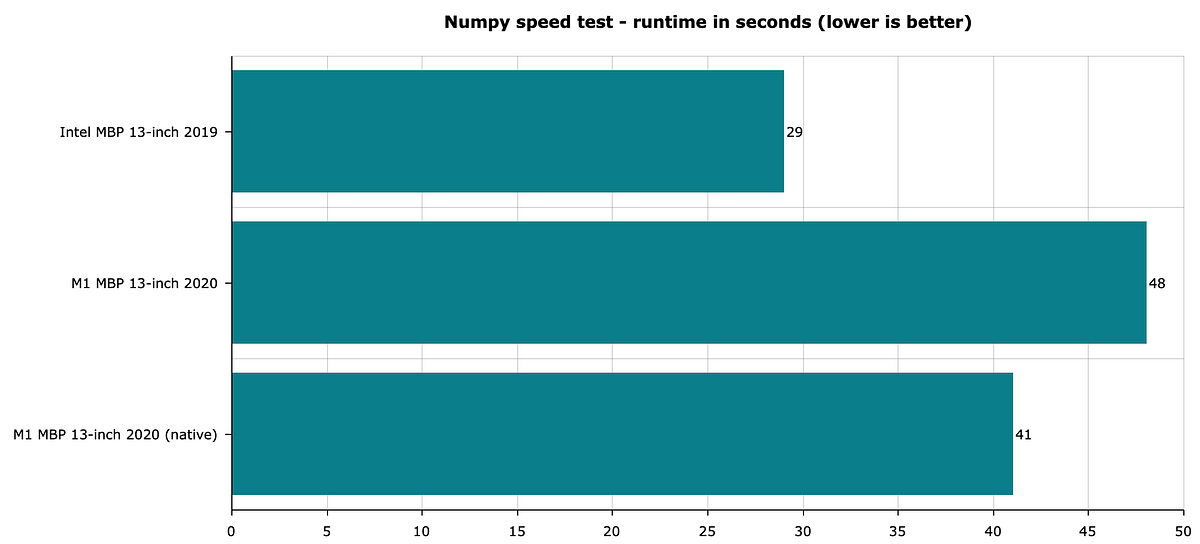Image 3 — Numpy speed test — lower is better (image by author)

Results obtained with Numpy are a bit strange, to say at least. It looks like Numpy runs faster on my 2019 Intel Mac for some reason. Maybe it’s due to some optimizations, but I can’t say for sure. If you know why, please don’t hesitate to share in the comment section.

Next, let’s compare the Pandas performance.

## Performance test — Pandas

Pandas benchmark is quite similar to the Python one. Identical operations were performed, but the results were combined into a single data frame.

• Create an empty data frame
• Assign it a column (`X`) of 100,000,000 random integers between 100 and 999
• Square every item in `X`
• Take a square root of every item in `X`
• Multiply corresponding squares and square roots
• Divide corresponding squares and square roots
• Perform an integer division of corresponding squares and square roots

Here’s the code snippet for the test:

``````import numpy as np
import pandas as pd
from datetime import datetime

time_start = datetime.now()

df = pd.DataFrame()
df['X'] = np.random.randint(low=100, high=999, size=100000000)
df['X_squared'] = df['X'].apply(lambda x: x**2)
df['X_sqrt'] = df['X'].apply(lambda x: x**0.5)
df['Mul'] = df['X_squared'] * df['X_sqrt']
df['Div'] = df['X_squared'] / df['X_sqrt']
df['Int_div'] = df['X_squared'] // df['X_sqrt']

time_end = datetime.now()
print(f'Total time = {(time_end - time_start).seconds} seconds')``````

And here are the results: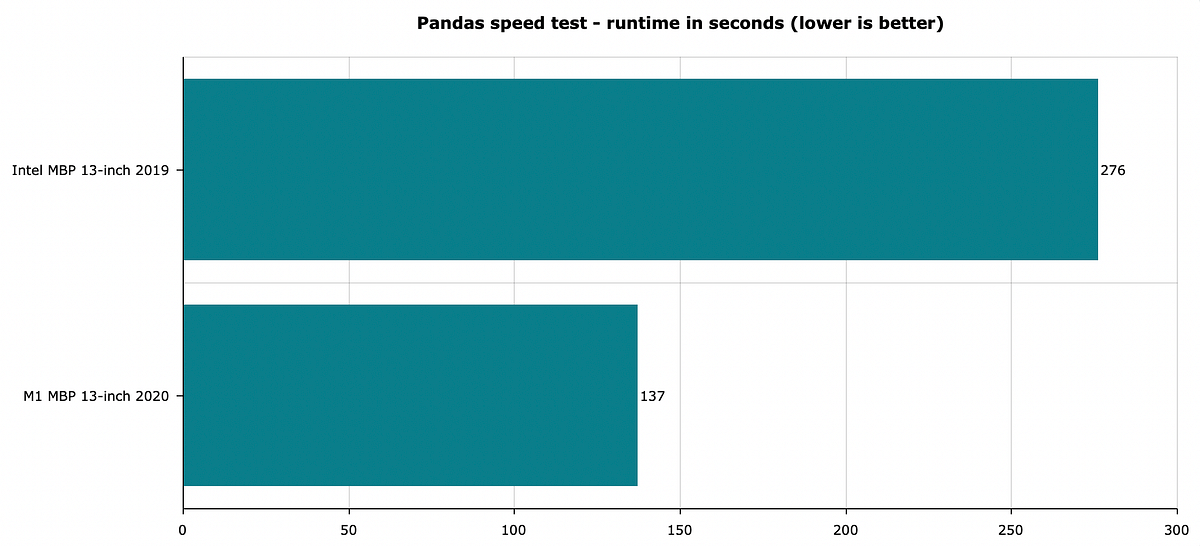Image 4 — Pandas speed test — lower is better (image by author)

As you can see, there’s no measurement for “native” Pandas, as I haven’t managed to install it. Still, Pandas on the M1 chip finished this benchmark two times faster.

## Performance test — Scikit-Learn

As with Pandas, I haven’t managed to install Scikit-Learn natively. You’ll only see comparisons between Intel MBP and M1 MBP running through the Rosseta 2 emulator.

Here’s a list of tasks performed in the benchmark:

• Get the dataset from the web
• Perform a train/test split
• Declare a Decision tree model and find optimal hyperparameters (2400 combinations + 5-fold cross-validation)
• Fit a model with optimal parameters

It’s a more or less standard model training procedure, disregarding testing out multiple algorithms, data preparation, and feature engineering.

Here’s the code snippet for the test:

``````from sklearn.tree import DecisionTreeClassifier
from sklearn.model_selection import train_test_split, GridSearchCV
from sklearn.metrics import accuracy_score, confusion_matrix

time_start = datetime.now()

# Dataset

# Train/Test split
X = iris.drop('species', axis=1)
y = iris['species']
X_train, X_test, y_train, y_test = train_test_split(X, y, test_size=0.25)
time_split = datetime.now()
print(f'Train/test split, runtime = {(time_split - time_start).seconds} seconds')

# Hyperparameter tuning
model = DecisionTreeClassifier()
params = {
'criterion': ['gini', 'entropy'],
'splitter': ['best', 'random'],
'max_depth': [1, 5, 10, 50, 100, 250, 500, 1000],
'min_samples_split': [2, 5, 10, 15, 20],
'min_samples_leaf': [1, 2, 3, 4, 5],
'max_features': ['auto', 'sqrt', 'log2']
}
clf = GridSearchCV(
estimator=model,
param_grid=params,
cv=5
)
clf.fit(X_train, y_train)
time_optim = datetime.now()
print(f'Hyperparameter optimization, runtime = {(time_optim - time_start).seconds} seconds')

best_model = DecisionTreeClassifier(**clf.best_params_)
best_model.fit(X_train, y_train)

time_end = datetime.now()
print()
print(f'TOTAL RUNTIME = {(time_end - time_start).seconds} seconds')``````

And here are the results: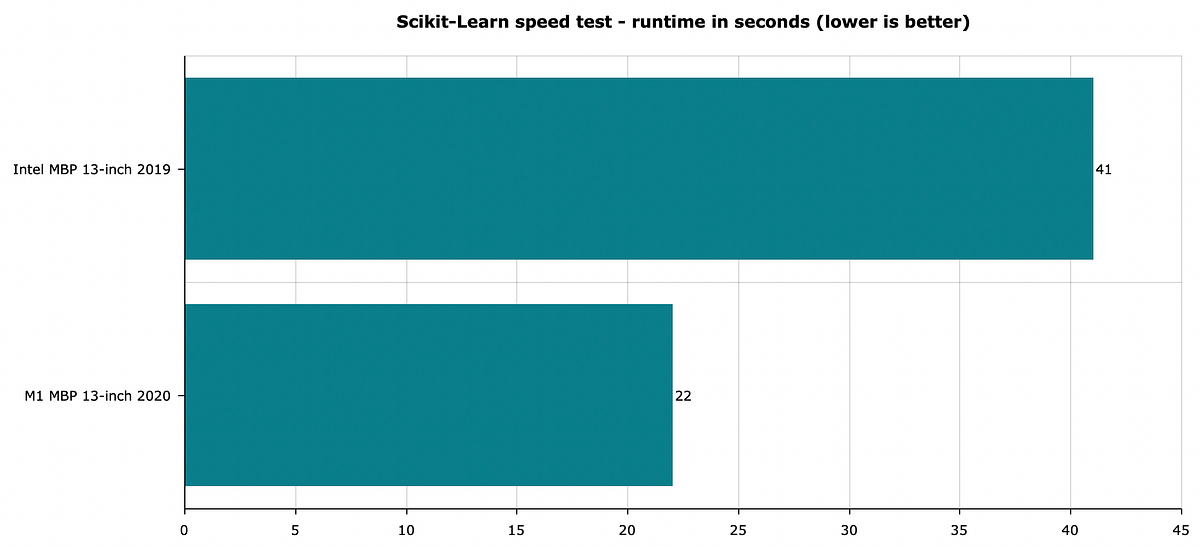Image 5 — Scikit-Learn speed test — lower is better (image by author)

The results convey the same information seen with Pandas — 2019 Intel i5 processor takes two times longer to finish the same task.

## Conclusion

The comparisons with the Intel-based 2019 Mac might be irrelevant to you. That’s great — you have the benchmark scripts so you can run the tests on your machine. Let me know if you do so — I’m eager to find out about your configuration and how it compares.

The new M1 chips are amazing, and the best is yet to come. This is only the first generation, after all. Macbooks aren’t machine learning workstations, but you’re still getting a good bang for the buck.

Deep learning benchmarks with TensorFlow are coming out next week, so stay tuned: